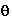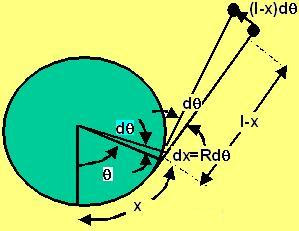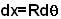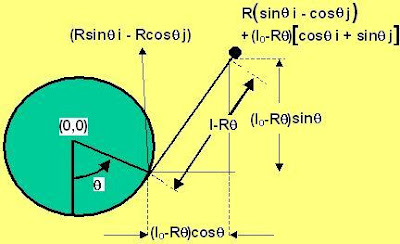Sunday, May 4, 2008

Irodov Problem 1.138The first thing to understand in this problem is that the only force that is acting on the disc is the tension and it always acts in a direction perpendicular to the direction of the velocity of the disc. In other words there is no tangential force acting on the disc at any point of time. Without any tangential force acting on the disc, the magnitude of the disc's velocity can never change. The tension in the string will only change the direction of motion of the disc. So throughout the disc's motion its speed will remain vo.

As the string wraps around the cylinder, the length of the string decreases and so the disc wraps around faster and faster. Suppose that at some instant of time the disc has wrapped around an angle of, this means that length of string that has wrapped around x is given by,At this point, the remaining length of the string will be l0-x.Suppose that the string has already wrapped around an angle of. Now suppose the string wraps around an additional angle of. The additional string length wrapped around dx is given by. During this time the string also turns an angle ofsince its always tangential to the cylinder. At this time the length of the string is l0 -x and so the disc will move a distance of. Suppose that the time elapsed is dt. We know that the disc was moving at a velocity v0 and so this means that,Equation (2) can also be deduced by a completely mathematical treatment as well. I will provide the completely mathematical way of getting (2) below, for the benefit of the readers.As seen the above figure when the string has wrapped an angle of, the location of the disc is given by,This is same as (2).

The disc will hit the cylinder when the entire length l0 has wrapped, in other words x=l0. Hence we have,1 comment:Anonymous said...

thank yo very much sir for this useful information.